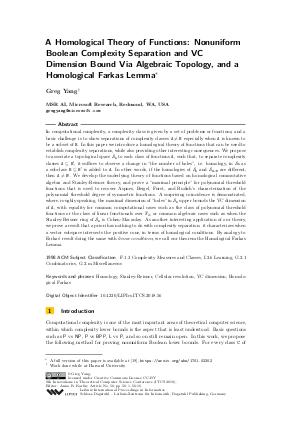Document# A Homological Theory of Functions: Nonuniform Boolean Complexity Separation and VC Dimension Bound Via Algebraic Topology, and a Homological Farkas Lemma

### Author Greg Yang## File

LIPIcs.ITCS.2018.56.pdf
• Filesize: 0.99 MB
• 16 pages

## Cite As

Greg Yang. A Homological Theory of Functions: Nonuniform Boolean Complexity Separation and VC Dimension Bound Via Algebraic Topology, and a Homological Farkas Lemma. In 9th Innovations in Theoretical Computer Science Conference (ITCS 2018). Leibniz International Proceedings in Informatics (LIPIcs), Volume 94, pp. 56:1-56:16, Schloss Dagstuhl - Leibniz-Zentrum für Informatik (2018)
https://doi.org/10.4230/LIPIcs.ITCS.2018.56

## Abstract

In computational complexity, a complexity class is given by a set of problems or functions, and a basic challenge is to show separations of complexity classes A != B especially when A is known to be a subset of B. In this paper we introduce a homological theory of functions that can be used to establish complexity separations, while also providing other interesting consequences. We propose to associate a topological space S_A to each class of functions A, such that, to separate complexity classes A from a superclass B', it suffices to observe a change in "the number of holes", i.e. homology, in S_A as a subclass B of B' is added to A. In other words, if the homologies of S_A and S_{A union B} are different, then A != B'. We develop the underlying theory of functions based on homological commutative algebra and Stanley-Reisner theory, and prove a "maximal principle" for polynomial threshold functions that is used to recover Aspnes, Beigel, Furst, and Rudich's characterization of the polynomial threshold degree of symmetric functions. A surprising coincidence is demonstrated, where, roughly speaking, the maximal dimension of "holes" in S_A upper bounds the VC dimension of A, with equality for common computational cases such as the class of polynomial threshold functions or the class of linear functionals over the finite field of 2 elements, or common algebraic cases such as when the Stanley-Reisner ring of S_A is Cohen-Macaulay. As another interesting application of our theory, we prove a result that a priori has nothing to do with complexity separation: it characterizes when a vector subspace intersects the positive cone, in terms of homological conditions. By analogy to Farkas' result doing the same with linear conditions, we call our theorem the Homological Farkas Lemma.
##### Keywords
• Homology
• Stanley-Reisner
• Cellular resolution
• VC dimension
• Homological Farkas

## Metrics

• Access Statistics
• Total Accesses (updated on a weekly basis)
0

## References

1. Scott Aaronson and Avi Wigderson. Algebrization: A new barrier in complexity theory. TOCT, 1(1):2:1-2:54, 2009. URL: http://dx.doi.org/10.1145/1490270.1490272.
2. James Aspnes, Richard Beigel, Merrick L. Furst, and Steven Rudich. The expressive power of voting polynomials. Combinatorica, 14(2):135-148, 1994. URL: http://dx.doi.org/10.1007/BF01215346.
3. Theodore P. Baker, John Gill, and Robert Solovay. Relativizations of the P =? NP question. SIAM J. Comput., 4(4):431-442, 1975. URL: http://dx.doi.org/10.1137/0204037.
4. Dave Bayer and Mike Stillman. Computation of Hilbert functions. Journal of Symbolic Computation, 14(1):31-50, 1992. URL: http://www.sciencedirect.com/science/article/pii/074771719290024X.
5. Michael Ben-Or. Lower bounds for algebraic computation trees (preliminary report). In David S. Johnson, Ronald Fagin, Michael L. Fredman, David Harel, Richard M. Karp, Nancy A. Lynch, Christos H. Papadimitriou, Ronald L. Rivest, Walter L. Ruzzo, and Joel I. Seiferas, editors, Proceedings of the 15th Annual ACM Symposium on Theory of Computing, 25-27 April, 1983, Boston, Massachusetts, USA, pages 80-86. ACM, 1983. URL: http://dx.doi.org/10.1145/800061.808735.
6. Anders Björner, László Lovász, and Andrew CC Yao. Linear decision trees: volume estimates and topological bounds. In Proceedings of the twenty-fourth annual ACM symposium on Theory of computing, pages 170-177. ACM, 1992. URL: http://dl.acm.org/citation.cfm?id=129730.
7. Stephen P. Boyd and Lieven Vandenberghe. Convex optimization. Cambridge University Press, Cambridge, UK ; New York, 2004.8. Joshua A. Grochow. Unifying known lower bounds via geometric complexity theory. Computational Complexity, 24(2):393-475, 2015. URL: http://dx.doi.org/10.1007/s00037-015-0103-x.
9. Maurice Herlihy and Nir Shavit. The topological structure of asynchronous computability. Journal of the ACM (JACM), 46(6):858-923, 1999. URL: http://dl.acm.org/citation.cfm?id=331529.
10. Michael Kearns and Umesh Vazirani. An Introduction to Computational Learning Theory. MIT Press, jan 1994.11. A. S. Kechris. Classical descriptive set theory. Number 156 in Graduate texts in mathematics. Springer-Verlag, New York, 1995.12. Tjalling C Koopmans and others. Activity analysis of production and allocation. Wiley, 1951.13. Yiannis N. Moschovakis. Descriptive set theory. Number v. 155 in Mathematical surveys and monographs. American Mathematical Society, Providence, R.I, 2nd ed edition, 2009.14. Ketan Mulmuley and Milind A. Sohoni. Geometric complexity theory I: an approach to the P vs. NP and related problems. SIAM J. Comput., 31(2):496-526, 2001. URL: http://dx.doi.org/10.1137/S009753970038715X.
15. Alexander A. Razborov and Steven Rudich. Natural proofs. J. Comput. Syst. Sci., 55(1):24-35, 1997. URL: http://dx.doi.org/10.1006/jcss.1997.1494.
16. Joseph J. Rotman. An introduction to algebraic topology. Number 119 in Graduate texts in mathematics. Springer-Verlag, New York, 1988.17. The Univalent Foundations Program. Homotopy Type Theory: Univalent Foundations of Mathematics. arXiv preprint arXiv:1308.0729, 2013. URL: https://arxiv.org/abs/1308.0729.
18. Klaus Weihrauch. Computable Analysis. Texts in Theoretical Computer Science. An EATCS Series. Springer Berlin Heidelberg, Berlin, Heidelberg, 2000. DOI: 10.1007/978-3-642-56999-9. URL: http://link.springer.com/10.1007/978-3-642-56999-9.
19. Greg Yang. A Homological Theory of Functions. arXiv:1701.02302 [cs, math], jan 2017. arXiv: 1701.02302. URL: http://arxiv.org/abs/1701.02302.
20. Andrew Chi-Chih Yao. Lower bounds for algebraic computation trees with integer inputs. SIAM J. Comput., 20(4):655-668, 1991. URL: http://dx.doi.org/10.1137/0220041.
21. Andrew Chi-Chih Yao. Decision tree complexity and betti numbers. In Frank Thomson Leighton and Michael T. Goodrich, editors, Proceedings of the Twenty-Sixth Annual ACM Symposium on Theory of Computing, 23-25 May 1994, Montréal, Québec, Canada, pages 615-624. ACM, 1994. URL: http://dx.doi.org/10.1145/195058.195414.
22. Günter M. Ziegler. Lectures on Polytopes, volume 152 of Graduate Texts in Mathematics. Springer New York, New York, NY, 1995. URL: http://link.springer.com/10.1007/978-1-4613-8431-1.
X

Feedback for Dagstuhl Publishing